Home

# NILPOTENT SUBSPACES AND NILPOTENT ORBITS

## Abstract

Let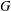$G$ be a semisimple complex algebraic group with Lie algebra$\mathfrak{g}$ . For a nilpotent$G$ -orbit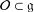${\mathcal{O}}\subset \mathfrak{g}$ , let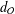$d_{{\mathcal{O}}}$ denote the maximal dimension of a subspace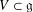$V\subset \mathfrak{g}$ that is contained in the closure of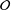${\mathcal{O}}$ . In this note, we prove that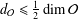$d_{{\mathcal{O}}}\leq {\textstyle \frac{1}{2}}\dim {\mathcal{O}}$ and this upper bound is attained if and only if${\mathcal{O}}$ is a Richardson orbit. Furthermore, if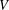$V$ is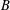$B$ -stable and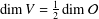$\dim V={\textstyle \frac{1}{2}}\dim {\mathcal{O}}$ , then$V$ is the nilradical of a polarisation of${\mathcal{O}}$ . Every nilpotent orbit closure has a distinguished$B$ -stable subspace constructed via an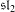$\mathfrak{sl}_{2}$ -triple, which is called the Dynkin ideal. We then characterise the nilpotent orbits${\mathcal{O}}$ such that the Dynkin ideal (1) has the minimal dimension among all$B$ -stable subspaces$\mathfrak{c}$ such that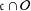$\mathfrak{c}\cap {\mathcal{O}}$ is dense in$\mathfrak{c}$ , or (2) is the only$B$ -stable subspace$\mathfrak{c}$ such that$\mathfrak{c}\cap {\mathcal{O}}$ is dense in$\mathfrak{c}$ .

## Footnotes

Hide All

The research of the first author was carried out at the IITP R.A.S. at the expense of the Russian Foundation for Sciences (project no. 14-50-00150). The second author is partially supported by the DFG priority programme SPP 1388 ‘Darstellungstheorie’ and by the Graduiertenkolleg GRK 1523 ‘Quanten- und Gravitationsfelder’.

## References

Hide All
MathJax
MathJax is a JavaScript display engine for mathematics. For more information see http://www.mathjax.org.

# NILPOTENT SUBSPACES AND NILPOTENT ORBITS

## Metrics

### Full text viewsFull text views reflects the number of PDF downloads, PDFs sent to Google Drive, Dropbox and Kindle and HTML full text views.

Total number of HTML views: 0
Total number of PDF views: 0 *Loading metrics...

### Abstract viewsAbstract views reflect the number of visits to the article landing page.

Total abstract views: 0 *Loading metrics...

* Views captured on Cambridge Core between <date>. This data will be updated every 24 hours.

Usage data cannot currently be displayed